Related Articles
Z-Buffer or Depth-Buffer method
• Difficulty Level : Hard
• Last Updated : 06 Mar, 2018

When viewing a picture containing non transparent objects and surfaces, it is not possible to see those objects from view which are behind from the objects closer to eye. To get the realistic screen image, removal of these hidden surfaces is must. The identification and removal of these surfaces is called as the Hidden-surface problem.

Z-buffer, which is also known as the Depth-buffer method is one of the commonly used method for hidden surface detection. It is an Image space method. Image space methods are based on the pixel to be drawn on 2D. For these methods, the running time complexity is the number of pixels times number of objects. And the space complexity is two times the number of pixels because two arrays of pixels are required, one for frame buffer and the other for the depth buffer.

The Z-buffer method compares surface depths at each pixel position on the projection plane. Normally z-axis is represented as the depth. The algorithm for the Z-buffer method is given below :

Algorithm :

```First of all, initialize the depth of each pixel.
i.e,  d(i, j) = infinite (max length)
Initialize the color value for each pixel
as c(i, j) = background color
for each polygon, do the following steps :

for (each pixel in polygon's projection)
{
find depth i.e, z of polygon
at (x, y) corresponding to pixel (i, j)

if (z < d(i, j))
{
d(i, j) = z;
c(i, j) = color;
}
}
```

Let’s consider an example to understand the algorithm in a better way. Assume the polygon given is as below :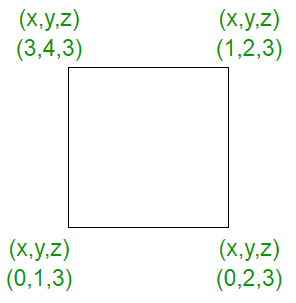In starting, assume that the depth of each pixel is infinite.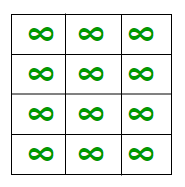As the z value i.e, the depth value at every place in the given polygon is 3, on applying the algorithm, the result is: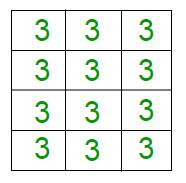Now, let’s change the z values. In the figure given below, the z values goes from 0 to 3.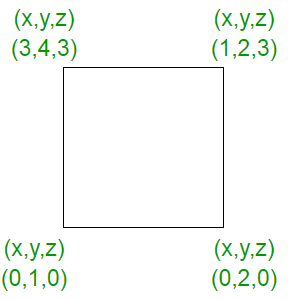In starting, the depth of each pixel will be infinite as :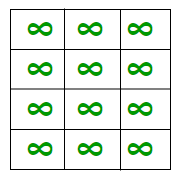Now, the z values generated on the pixel will be different which are as shown below :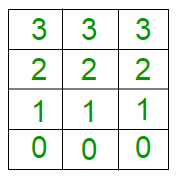Therefore, in the Z buffer method, each surface is processed separately one position at a time across the surface. After that the depth values i.e, the z values for a pixel are compared and the closest i.e, (smallest z) surface determines the color to be displayed in frame buffer. The z values, i.e, the depth values are usually normalized to the range [0, 1]. When the z = 0, it is known as Back Clipping Pane and when z = 1, it is called as the Front Clipping Pane.

In this method, 2 buffers are used :

1. Frame buffer
2. Depth buffer

Calculation of depth :
As we know that the equation of the plane is :

```ax + by + cz + d = 0, this implies

z = -(ax + by + d)/c, c!=0
```

Calculation of each depth could be very expensive, but the computation can be reduced to a single add per pixel by using an increment method as shown in figure below :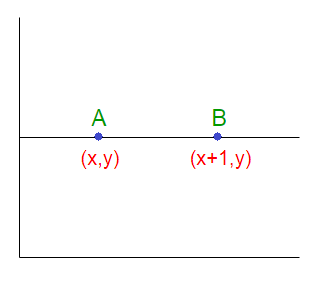Let’s denote the depth at point A as Z and at point B as Z’. Therefore :

```AX + BY + CZ + D = 0 implies

Z = (-AX - BY - D)/C  ------------(1)

Similarly, Z' = (-A(X + 1) - BY -D)/C   ----------(2)

Hence from (1) and (2), we conclude :

Z' = Z - A/C  ------------(3)
```

Hence, calculation of depth can be done by recording the plane equation of each polygon in the (normalized) viewing coordinate system and then using the incremental method to find the depth Z.
So, to summarize, it can be said that this approach compares surface depths at each pixel position on the projection plane. Object depth is usually measured from the view plane along the z-axis of a viewing system.
Example :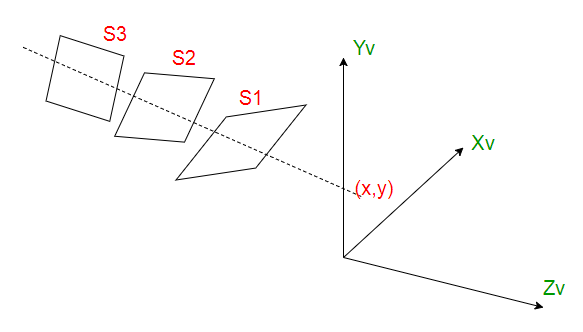Let S1, S2, S3 are the surfaces. The surface closest to projection plane is called visible surface. The computer would start (arbitrarily) with surface 1 and put it’s value into the buffer. It would do the same for the next surface. It would then check each overlapping pixel and check to see which one is closer to the viewer and then display the appropriate color. As at view-plane position (x, y), surface S1 has the smallest depth from the view plane, so it is visible at that position.

Points to remember :
1) Z buffer method does not require pre-sorting of polygons.
2) This method can be executed quickly even with many polygons.
3) This can be implemented in hardware to overcome the speed problem.
4) No object to object comparison is required.
5) This method can be applied to non-polygonal objects.
6) Hardware implementation of this algorithm are available in some graphics workstations.
7) The method is simple to use and does not require additional data structure.
8) The z-value of a polygon can be calculated incrementally.
9) Cannot be applied on transparent surfaces i.e, it only deals with opaque surfaces. For ex :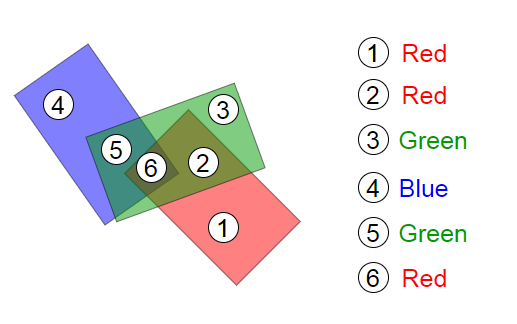10) If only a few objects in the scene are to be rendered, then this method is less attractive because of additional buffer and the overhead involved in updating the buffer.
11) Wastage of time may occur because of drawing of hidden objects.

My Personal Notes arrow_drop_up